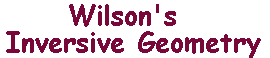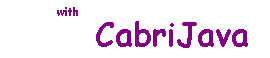Proof of the Common Inverses Theorem

 The Common Inverses Theorem. If L and M are non-intersecting i-lines, then there exists a unique pair of points {A,B} inverse with respect to both L and M. Proof Since all extended lines pass through Ñ, L and M cannot both be extended lines. Suppose that L is an extended line. Then M must be a circle. Let it have centre P and radius r. If A and B are inverse with respect to L, they must lie on a line perpendicular to L. Also, they must lie on opposite sides of L. Say that A lies on the same side as M. If A and B are inverse with respect to M, they must lie on a line through P, and on the same side as P. Also one must lie inside M. Since B lies on the opposite side of L, A is inside M. Then L, M, A, B and P are as in the picture on the right, and Q is the foot of the perpendicular from P to L. Suppose that A and B are inverse with respect to L, so QA = QB. Let N denote the circle on AB as diameter, and suppose it meets M at R. Then QA = QB = QR. Now, A and B are inverse with respect to M if and only if PA.PB = r2 = PR2. i.e. PR2 = PA.PB = (PQ-QA).(PQ+QB) = PQ2-QR2 (as QA = QB = QR). Thus, A and B are common inverses if and only if